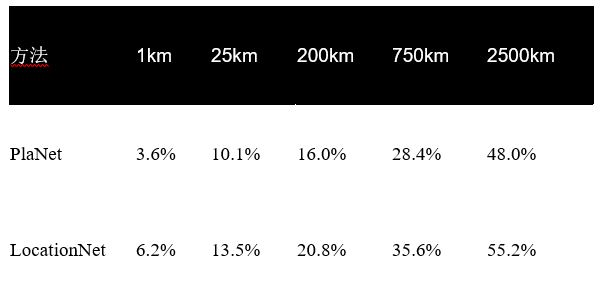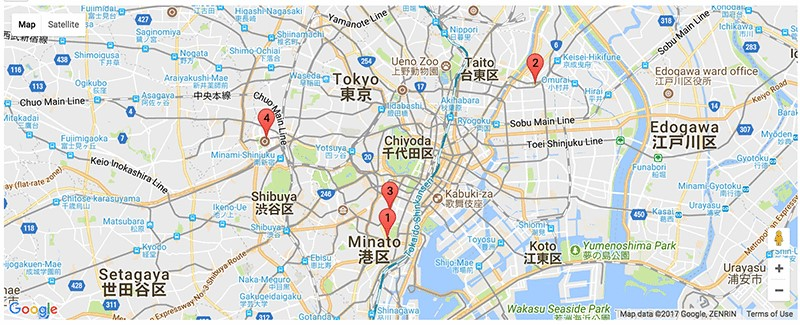使用 AWS EC2 上的 Apache MXNet 和 Multimedia Commons 数据集来估计图像位置

我们的方法

Weyand 等人近期的作品提出图像定位是一个分类问题。在这种方法中，作者将地球表面细分为数千个地理单元格，并利用带地理标记的图像训练了深层神经网路。有关他们的试验更通俗的描述，请参阅该文章

 LocationNet PlaNet 数据集来源 Multimedia Commons 从网络抓取的图像 训练集 3390 万 9100 万 验证 180 万 3400 万 S2 单元分区 t1=5000, t2=500 → 15,527 个单元格 t1=10,000, t2=50 → 26,263 个单元格 模型 ResNet-101 GoogleNet 优化 使用动量和 LR 计划的 SGD Adagrad 训练时间 采用 16 个 NVIDIA K80 GPU (p2.16xlarge EC2 实例) 时为 9 天 12 个训练周期 采用 200 个 CPU 内核时为两个半月 框架 MXNet DistBelief 测试集 Placing Task 2016 测试集 (150 万张 Flickr 图像) 230 万张有地理标记的 Flickr 图像

LocationNet 会在 MXNet Model Zoo 中公开分享。

下载 LocationNet

LocationNet 包括两部分：一个包含模型定义的 JSON 文件和一个包含参数的二进制文件。我们从 S3 加载必要的软件包并下载文件。

import os

import urllib

import mxnet as mx

import logging

import numpy as np

from skimage import io, transform

from collections import namedtuple

from math import radians, sin, cos, sqrt, asin

path = 'https://s3.amazonaws.com/mmcommons-tutorial/models/'

model_path = 'models/'

if not os.path.exists(model_path):

os.mkdir(model_path)

urllib.urlretrieve(path+'RN101-5k500-symbol.json', model_path+'RN101-5k500-symbol.json')

urllib.urlretrieve(path+'RN101-5k500-0012.params', model_path+'RN101-5k500-0012.params')

# Load the pre-trained model

prefix = "models/RN101-5k500"

mod = mx.mod.Module(symbol=sym, context=mx.gpu())

mod.bind([('data', (1,3,224,224))], for_training=False)
mod.set_params(arg_params, aux_params, allow_missing=True)

grids.txt 文件包含用于训练模型的地理单元格。

urllib.urlretrieve('https://raw.githubusercontent.com/multimedia-berkeley/tutorials/master/grids.txt','grids.txt')

grids = []

with open('grids.txt', 'r') as f:

for line in f:

line = line.strip().split('\t')

lat = float(line)

lng = float(line)

grids.append((lat, lng))

def distance(p1, p2):

R = 6371 # Earth radius in km

lat1, lng1, lat2, lng2 = map(radians, (p1, p1, p2, p2))

dlat = lat2 - lat1

dlng = lng2 - lng1

a = sin(dlat * 0.5) ** 2 + cos(lat1) * cos(lat2) * (sin(dlng * 0.5) ** 2)
return 2 * R * asin(sqrt(a))

# mean image for preprocessing

mean_rgb = np.array([123.68, 116.779, 103.939])

mean_rgb = mean_rgb.reshape((3, 1, 1))

def PreprocessImage(path, show_img=False):

# We crop image from center to get size 224x224.

short_side = min(img.shape[:2])

yy = int((img.shape - short_side) / 2)

xx = int((img.shape - short_side) / 2)

crop_img = img[yy : yy + short_side, xx : xx + short_side]

resized_img = transform.resize(crop_img, (224,224))

if show_img:

io.imshow(resized_img)

# convert to numpy.ndarray

sample = np.asarray(resized_img) * 256

# swap axes to make image from (224, 224, 3) to (3, 224, 224)

sample = np.swapaxes(sample, 0, 2)

sample = np.swapaxes(sample, 1, 2)

# sub mean

normed_img = sample - mean_rgb

normed_img = normed_img.reshape((1, 3, 224, 224))
return [mx.nd.array(normed_img)]

评估并比较模型

IM2GPS 测试集结果使用 URL 估算图像的地理位置

Batch = namedtuple('Batch', ['data'])

def predict(imgurl, prefix='images/'):

imgname = imgurl.split('/')[-1]

batch = PreprocessImage(prefix + imgname, True)

#predict and show top 5 results

mod.forward(Batch(batch), is_train=False)

prob = mod.get_outputs().asnumpy()

pred = np.argsort(prob)[::-1]

result = list()

for i in range(5):

pred_loc = grids[int(pred[i])]

res = (i+1, prob[pred[i]], pred_loc)

print('rank=%d, prob=%f, lat=%s, lng=%s' \

% (i+1, prob[pred[i]], pred_loc, pred_loc))

result.append(res)

return result

if not os.path.exists(img_directory):

os.mkdir(img_directory)

imgname = imgurl.split('/')[-1]

filepath = os.path.join(img_directory, imgname)

if not os.path.exists(filepath):

filepath, _ = urllib.urlretrieve(imgurl, filepath)

statinfo = os.stat(filepath)

return filepath

#download and predict geo-location of an image of Tokyo Tower

url = 'https://farm5.staticflickr.com/4275/34103081894_f7c9bfa86c_k_d.jpg'

rank=1, prob=0.139923, lat=35.6599344486, lng=139.728919109

rank=2, prob=0.095210, lat=35.6546613641, lng=139.745685815

rank=3, prob=0.042224, lat=35.7098435803, lng=139.810458528

rank=4, prob=0.032602, lat=35.6641725688, lng=139.746648114

rank=5, prob=0.023119, lat=35.6901996892, lng=139.692857396

在 Jupyter Notebook 上使用 Google Maps 直观显示结果

import gmaps

gmaps.configure(api_key="") # Fill in with your API key

fig = gmaps.figure()

for i in range(len(result)):

marker = gmaps.marker_layer([result[i]], label=str(i+1))

fig